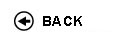Politecnico di Torino
Academic Year 2009/10
01BXTDR, 01BXTAX, 01BXTJA
Probability and statistics
1st degree and Bachelor-level of the Bologna process in Mechanical Engineering - Vercelli
1st degree and Bachelor-level of the Bologna process in Civil Engineering - Vercelli
1st degree and Bachelor-level of the Bologna process in Electronic And Computer Engineering - Vercelli
 Teacher Status SSD Les Ex Lab Tut Years teaching Durando Giovanni 30 20 0 20 1
 SSD CFU Activities Area context MAT/06 5 A - Di base Matematica, informatica e statistica
 Objectives of the course Provide basic knowledge on probability theory and statistics. Expected skills To be able to solve problems of probability theory making use of the concepts of discrete and continuous random variables, joint and conditional probability. Computing generating functions of common discrete and continuous probability distributions: binomial, Poisson, exponential. Extracting statistical properties from a dataset. Knowning the typical graphical representations of the statistical properties of a dataset. Performing hypothesis testing. Prerequisites Calculus I, II Syllabus Method of least squares. Covariance, correlation coefficient. Probability axioms: positivity, normalization, summability. Classical probability. Discrete probability, combinatorial calculus. Conditional probability. Bayes' formula. Independent events. Discrete random variables. Probability distribution of a random variable. Probability distribution of Bernoulli's, binomial, geometric random variables. Expectation value, variance. Variance and covariance. Chebichev inequality. Expectation value and variance of the sample average, law of large numbers. Poisson's law of rare events. Generating function of the moments. Continuous random variables. Jont and marginal probability distribution. Sum of independent random variables. The exponential and Gamma laws. Sum of normal random variables. Central limit theorem. Normality test, normal probability plot. Confidence intervals for mean and variance, chi-square and Student laws. Hypothesis testing for the mean with known and unknown variance. Laboratories and/or exercises Exercises about the arguments of the course are discussed and solved in the class. Bibliography Devore, 'Probability and Statistics for Engineering and the Sciences', Duxbury Press Revisions / Exam The exam consists of written exercises and questions about the arguments discussed in the course. Programma definitivo per l'A.A.2009/10© Politecnico di Torino
Corso Duca degli Abruzzi, 24 - 10129 Torino, ITALY